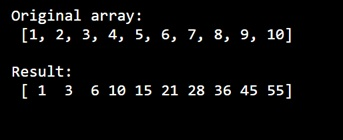# NumPy: How to make a moving(growing) sum of table contents without a for loop?

Learn, how to make a moving(growing) sum of table contents without a for loop in Python NumPy?
Submitted by Pranit Sharma, on January 02, 2023

NumPy is an abbreviated form of Numerical Python. It is used for different types of scientific operations in python. Numpy is a vast library in python which is used for almost every kind of scientific or mathematical operation. It is itself an array which is a collection of various methods and functions for processing the arrays.

## Python NumPy - Moving (growing) sum of table contents without a for loop

Suppose we are given a NumPy array and we need to create an array of moving sum.

For this purpose, we will use numpy.cumsum() method inside which we will pass the array as an argument.

This method returns the cumulative sum of the elements along a given axis. It takes an input array and an axis along which the cumulative sum is computed. The default (None) is to compute the cumsum over the flattened array.

Let us understand with the help of an example,

## Python code to make a moving(growing) sum of table contents without a for loop

```# Import numpy
import numpy as np

# Creating a numpy array
arr = [1,2,3,4,5,6,7,8,9,10]

# Display original array
print("Original array:\n",arr,"\n")

# Calculating cumsum
res = np.cumsum(arr)

# Display Result
print("Result:\n",res,"\n")
```

Output: